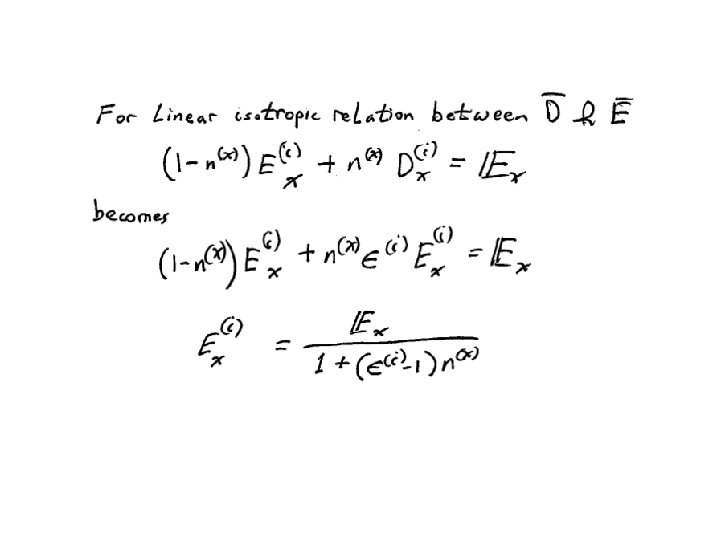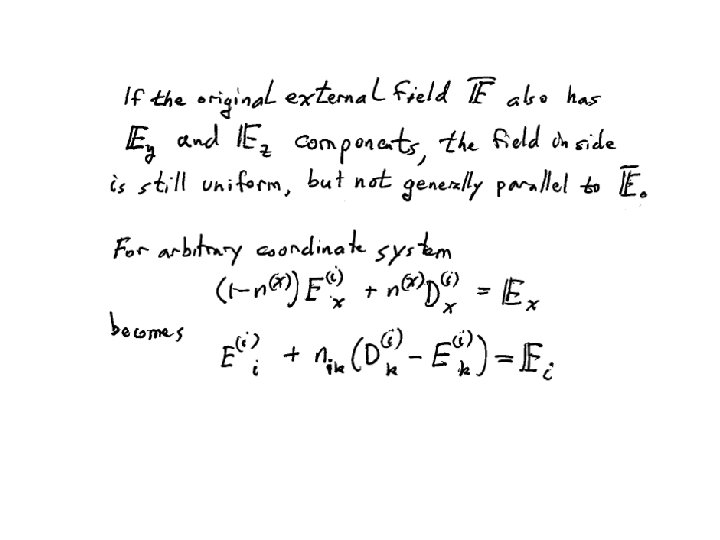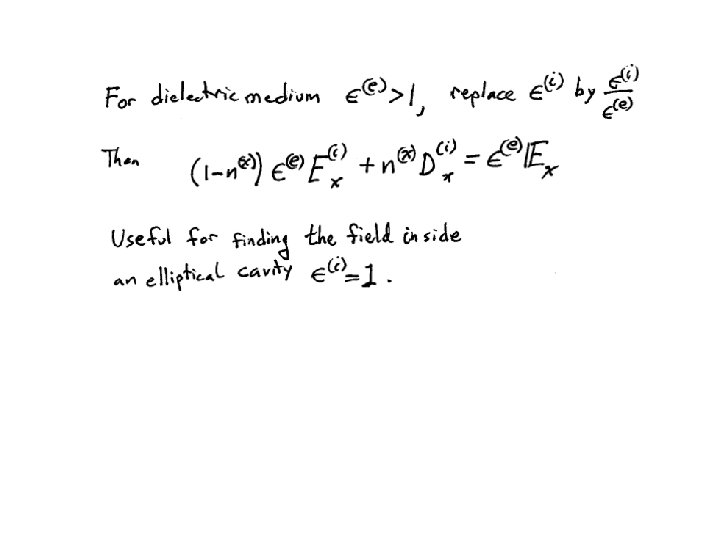# Dielectric Ellipsoid Section 8 Dielectric sphere in a

• Slides: 24Dielectric Ellipsoid Section 8Dielectric sphere in a uniform external electric field Field that would exist without the sphere Put the origin at the center of the sphere.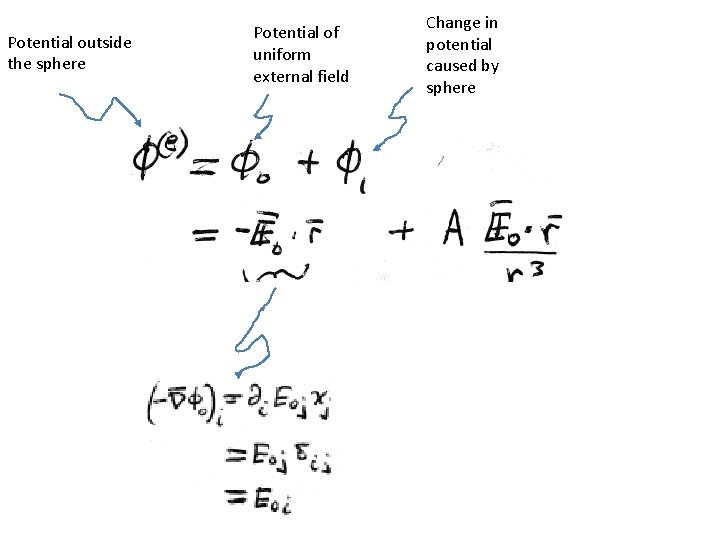Potential outside the sphere Potential of uniform external field Change in potential caused by sphere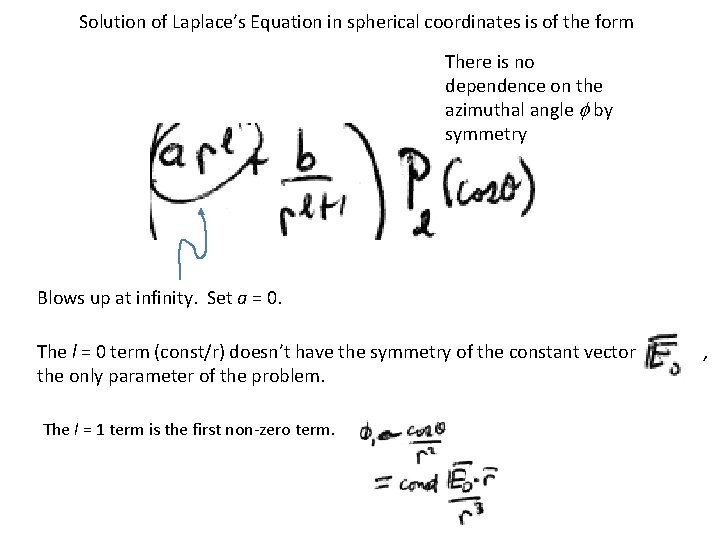Solution of Laplace’s Equation in spherical coordinates is of the form There is no dependence on the azimuthal angle f by symmetry Blows up at infinity. Set a = 0. The l = 0 term (const/r) doesn’t have the symmetry of the constant vector the only parameter of the problem. The l = 1 term is the first non-zero term. ,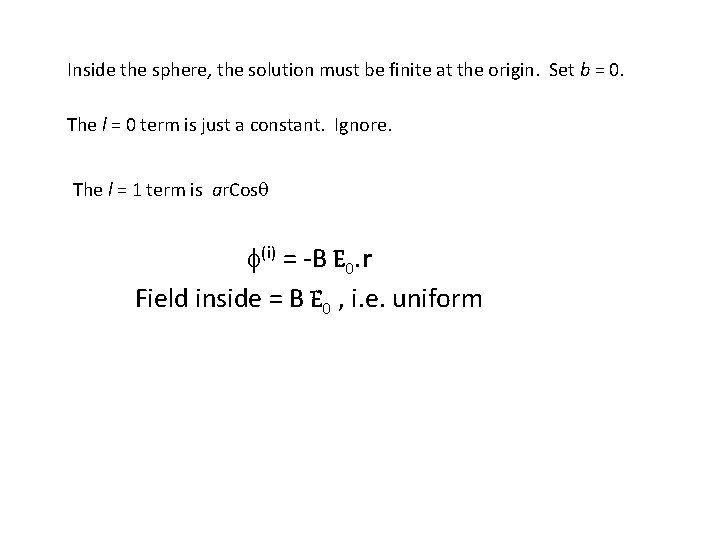Inside the sphere, the solution must be finite at the origin. Set b = 0. The l = 0 term is just a constant. Ignore. The l = 1 term is ar. Cosq f(i) = -B E 0. r Field inside = B E 0 , i. e. uniformBoundary condition on potentialNormal component of induction is continuousField inside dielectric sphere The sketch is for E(e) is similar to that of a conducting sphere in a uniform field.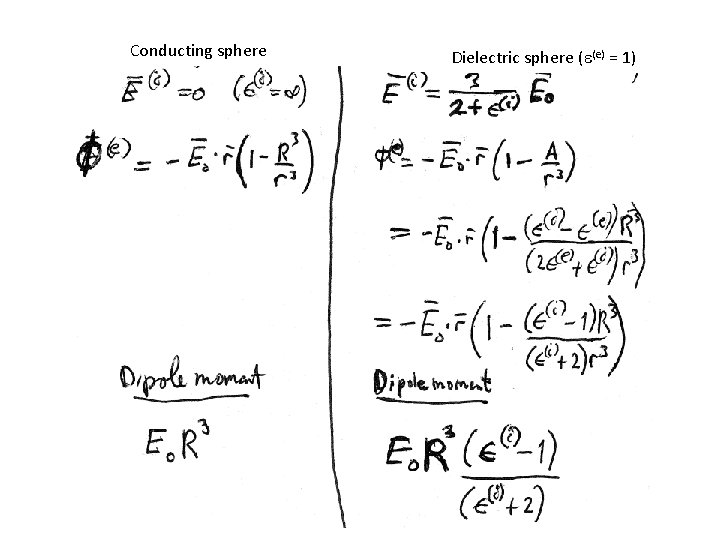Conducting sphere Dielectric sphere (e(e) = 1)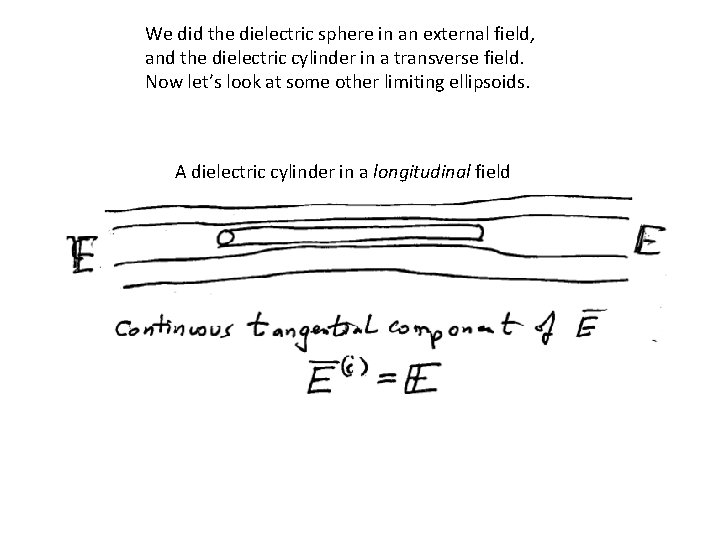We did the dielectric sphere in an external field, and the dielectric cylinder in a transverse field. Now let’s look at some other limiting ellipsoids. A dielectric cylinder in a longitudinal fieldFlat dielectric plate in a normal external fieldTheorem Whatever the ratio of the semiaxes a, b, c, the internal field of a dielectric ellipsoid placed in a uniform external field is uniform. Solution already found for the external field of the conducting ellipsoid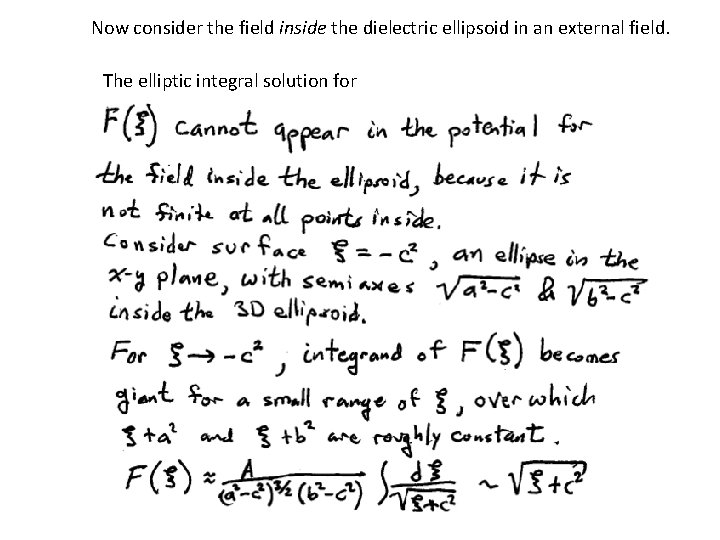Now consider the field inside the dielectric ellipsoid in an external field. The elliptic integral solution forThe solution we discarded for the external field of the conducting ellipsoid